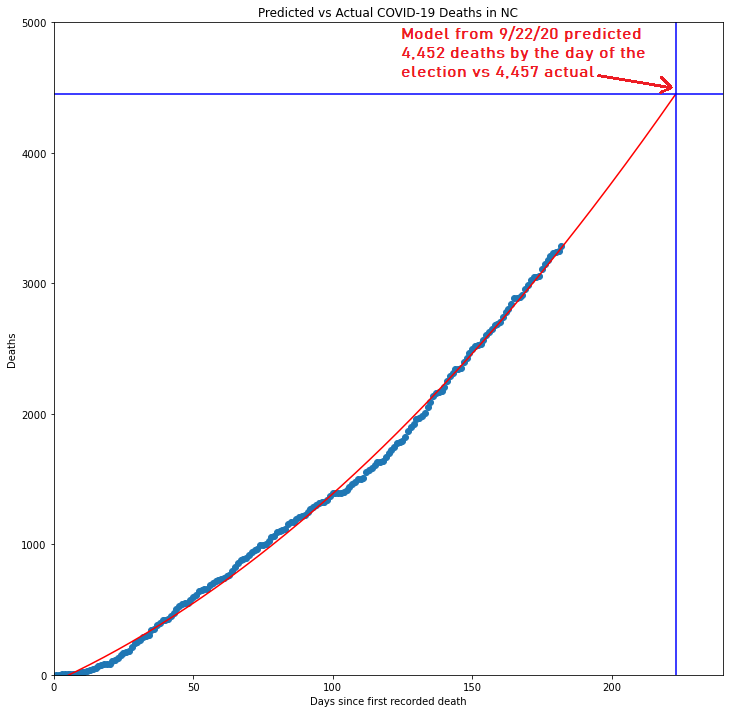# Forecasting Deaths From COVID-19 in North Carolina up to the End of 2020Photo by Ruthson Zimmerman on Unsplash

# ContextMatplotlib scatter plot of the actual death data up to 9/22 and a 41-day forecast leading up to the election today

# Updating the model with new data

`# Loading in most recent data updated as of today Nov. 3rdNCcovidNew = pd.read_excel('NCcovidNew.xlsx', sheet_name='Sheet 1')`
`date = NCcovidNew['Date']y = NCcovidNew['NC Deaths']date.shape # 224 days of data# Reshpaing the data x=np.linspace(224,1,224)x=np.reshape(x,(224,1))y=np.array(y)y=np.reshape(y,(224,1))`

# Refitting the model

`reg = linear_model.LinearRegression() # Constructing SKlearn modelreg.fit()`
`poly_f=PolynomialFeatures(degree=2,include_bias=False) # Instantiating quadratic termX_poly=poly_f.fit_transform(x) # Applying quadratic transformationreg.fit(X_poly,y) # Fitting transformed data to model# Assigning variables to the model's parameter estimatesa=reg.intercept_b=reg.coef_c=reg.coef_print(“a (Intercept term) =”,a)print(“b (Coefficient 1) =”,b)print(“c (Coefficient 2) =”,c)`

# Putting it all together

`plt.figure(figsize=(14,14))plt.scatter(x,y)x=np.linspace(282,1,282)x=np.reshape(x,(282,1))plt.xlim(0, 290)plt.ylim(0,8000)plt.plot(x, a + b*x+c*np.power(x,2), "r")plt.title('Predicted vs Actual COVID-19 Deaths in NC')plt.xlabel('Days since first recorded death')plt.ylabel('Deaths')plt.axvline(x=224+58,color='b') # Blue vertical line corresponds with Dec. 31stplt.axhline(y=a + b*(224+58) + c*(224+58)**2,color='b') plt.axhline(y=a + b*(224) + c*(224)**2,color='g') # Corresponds with today's death total of 4,457`

# Prediction

`deathsByNewYears = a + b*(224+58) + c*(224+58)**2 # Forecasting 58 days into the future from todayprint("Predicted number of deaths on Dec. 31st:",int(deathsByNewYears))`

# Here in North Carolina, the rate of positive tests have also picked back up in recent weeks